# AC Network Analysis

## Network Analysis Techniques

• #### Question 1

 Don’t just sit there! Build something!!

Learning to mathematically analyze circuits requires much study and practice. Typically, students practice by working through lots of sample problems and checking their answers against those provided by the textbook or the instructor. While this is good, there is a much better way.

You will learn much more by actually building and analyzing real circuits, letting your test equipment provide the “answers” instead of a book or another person. For successful circuit-building exercises, follow these steps:

1. Carefully measure and record all component values prior to circuit construction.
2. Draw the schematic diagram for the circuit to be analyzed.
3. Carefully build this circuit on a breadboard or other convenient medium.
4. Check the accuracy of the circuit’s construction, following each wire to each connection point, and verifying these elements one-by-one on the diagram.
5. Mathematically analyze the circuit, solving for all voltage and current values.
6. Carefully measure all voltages and currents, to verify the accuracy of your analysis.
7. If there are any substantial errors (greater than a few percent), carefully check your circuit’s construction against the diagram, then carefully re-calculate the values and re-measure.

For AC circuits where inductive and capacitive reactances (impedances) are a significant element in the calculations, I recommend high quality (high-Q) inductors and capacitors, and powering your circuit with low frequency voltage (power-line frequency works well) to minimize parasitic effects. If you are on a restricted budget, I have found that inexpensive electronic musical keyboards serve well as “function generators” for producing a wide range of audio-frequency AC signals. Be sure to choose a keyboard “voice” that closely mimics a sine wave (the “panflute” voice is typically good), if sinusoidal waveforms are an important assumption in your calculations.

As usual, avoid very high and very low resistor values, to avoid measurement errors caused by meter “loading”. I recommend resistor values between 1 kΩ and 100 kΩ.

One way you can save time and reduce the possibility of error is to begin with a very simple circuit and incrementally add components to increase its complexity after each analysis, rather than building a whole new circuit for each practice problem. Another time-saving technique is to re-use the same components in a variety of different circuit configurations. This way, you won’t have to measure any component’s value more than once.

• #### Question 2

This phase-shifting bridge circuit is supposed to provide an output voltage with a variable phase shift from -45o (lagging) to 45o (leading), depending on the position of the potentiometer wiper: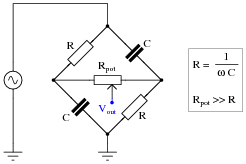Suppose, though, that the output signal is stuck at -45o lagging the source voltage, no matter where the potentiometer is set. Identify a likely failure that could cause this to happen, and explain why this failure could account for the circuit’s strange behavior.

• #### Question 3

Complex number arithmetic makes possible the analysis of AC circuits using (almost) the exact same Laws that were learned for DC circuit analysis. The only bad part about this is that doing complex-number arithmetic by hand can be very tedious. Some calculators, though, are able to add, subtract, multiply, divide, and invert complex quantities as easy as they do scalar quantities, making this method of AC circuit analysis relatively easy.

This question is really a series of practice problems in complex number arithmetic, the purpose being to give you lots of practice using the complex number facilities of your calculator (or to give you a lot of practice doing trigonometry calculations, if your calculator does not have the ability to manipulate complex numbers!).

 $$(5+j6) + (2-j1)=$$ $$(10 − j8) + (4 − j3) =$$ $$(−3 + j0) + (9 − j12) =$$

 $$(3 + j5) − (0 − j9) =$$ $$(25 − j84) − (4 − j3) =$$ $$(−1500 + j40) + (299 − j128) =$$

 $$(25\angle15^0)+(10\angle74^0)=$$ $$(1000\angle43^0)+(1200\angle-20^0)=$$ $$(522\angle71^0)-(85\angle30^0)=$$

Multiplication and division:

 $$(25\angle15^0)x(12\angle10^0)=$$ $$(1\angle25^0)x(500\angle-30^0)=$$ $$(522\angle71^0)x(33\angle9^0)=$$

 $$\frac {10\angle-80^0}{1\angle0^0}=$$ $$\frac {25\angle120^0}{3.5\angle-55^0}=$$ $$\frac {-66\angle67^0}{8\angle-42^0}=$$

 $$(3 + j5) × (2 − j1) =$$ $$(10 − j8) × (4 − j3) =$$ $$\frac {(3+j4)}{12-j2}=$$

Reciprocation:

$$\frac {1}{(15\angle60^0)}=$$

$$\frac {1}{(750\angle-38^0)}=$$

$$\frac {1}{(10+j3)}=$$

$$\frac {1}{\frac{1}{15\angle45^0}+\frac{1}{92\angle-25^0}}=$$

$$\frac {1}{\frac{1}{1200\angle73^0}+\frac{1}{574\angle21^0}}=$$

$$\frac {1}{\frac{1}{23k\angle-67^0}+\frac{1}{10k\angle-81^0}}=$$

$$\frac {1}{\frac{1}{100\angle-34^0}+\frac{1}{80\angle19^0}+\frac{1}{70\angle10}}=$$

$$\frac {1}{\frac{1}{89k\angle-5^0}+\frac{1}{15k\angle33^0}+\frac{1}{9.35k\angle45}}=$$

$$\frac {1}{\frac{1}{512\angle-34^0}+\frac{1}{1k\angle-25^0}+\frac{1}{942\angle-20}+\frac{1}{2.2k\angle44^0}}=$$

• #### Question 4

Is it safe to close the breaker between these two alternators if their output frequencies are different? Explain why or why not.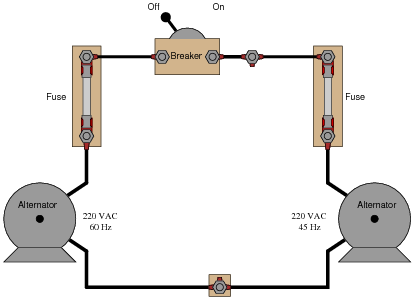• #### Question 5

Given the output voltages of the two alternators, it is not safe to close the breaker. Explain why.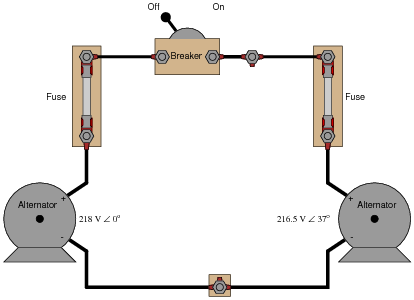• #### Question 6

A remote speaker for an audio system is connected to the amplifier by means of a long, 2-conductor cable: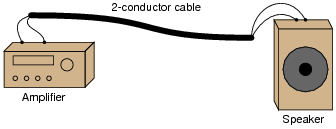This system may be schematically modeled as an AC voltage source connected to a load resistor: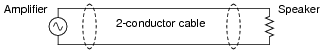Suppose we decided to use the 2-conductor cable for more than just conveying an audio (AC) signal - we want to use it to carry DC power as well to energize a small lamp. However, if we were to simply connect the DC power source in parallel with the amplifier output at one end, and the lamp in parallel with the speaker at the other, bad things would happen: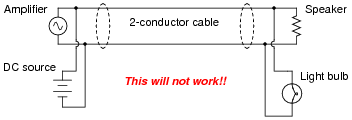If we were to connect the components together as shown above, the DC power source will likely damage the amplifier by being directly connected to it, the speaker will definitely be damaged by the application of significant DC voltage to its coil, and the light bulb will waste audio power by acting as a second (non-audible) load. Suffice to say, this is a bad idea.

Using inductors and capacitors as “filtering” components, though, we can make this system work: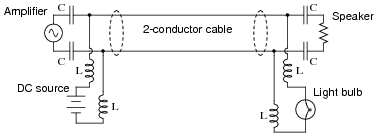Apply the Superposition Theorem to this circuit to demonstrate that the audio and DC signals will not interfere with each other as they would if directly connected. Assume that the capacitors are of such large value that they present negligible impedance to the audio signal (ZC ≈ 0 Ω) and that the inductors are sufficiently large that they present infinite impedance to the audio signal (ZL ≈ ∞).

• #### Question 7

Explain why this bridge circuit can never achieve balance: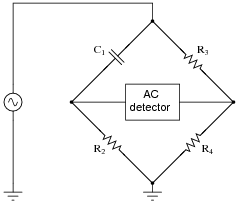• #### Question 8

Calculate the impedance value necessary to balance this AC bridge, expressing your answer in both polar and rectangular forms:• #### Question 9

Calculate the impedance value necessary to balance this AC bridge, expressing your answer in both polar and rectangular forms:Also, describe what sort of device might be appropriate to serve as a “null detector” to indicate when bridge balance has been achieved, and where this device would be connected to in the bridge circuit.

• #### Question 10

An AC bridge circuit commonly used to make precision measurements of inductors is the Maxwell-Wien bridge. It uses a combination of standard resistors and capacitors to “balance out” the inductor of unknown value in the opposite arm of the bridge: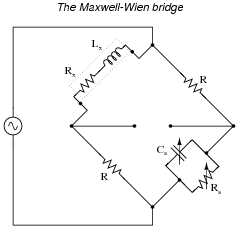Suppose this bridge circuit balances when Cs is adjusted to 120 nF and Rs is adjusted to 14.25 kΩ. If the source frequency is 400 Hz, and the two fixed-value resistors are 1 kΩ each, calculate the inductance (Lx) and resistance (Rx) of the inductor being tested.

• #### Question 11

Electrical engineers often represent impedances in rectangular form for the sake of algebraic manipulation: to be able to construct and manipulate equations involving impedance, in terms of the components’ fundamental values (resistors in ohms, capacitors in farads, and inductors in henrys).

For example, the impedance of a series-connected resistor (R) and inductor (L) would be represented as follows, with angular velocity (ω) being equal to 2 πf:

 Z = R + j ωL

Using the same algebraic notation, represent each of the following complex quantities:

Impedance of a single capacitor (C) =
Impedance of a series resistor-capacitor (R, C) network =
Admittance of a parallel inductor-resistor (L, R) network =
Admittance of a parallel resistor-capacitor (R, C) network =
• #### Question 12

Mathematical analysis of the Maxwell-Wien bridge is as follows: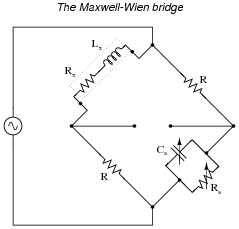$$Z_x=R_x+jwL_x$$ Impedance of unknown inductance/resistance arm

 $$Z_s= \frac{1}{\frac{1}{R_s}+\frac{1}{-j\frac{1}{wC_s}}}$$ Impedance of standard capacitance/resistance arm

 $$Y_s= \frac{1}{R_s}+jwC_s$$ Admittance of standard capacitance/resistance arm

 $$\frac{Z_x}{Z_R}=\frac{Z_R}{Z_s} \ \ \ \ \ \ \ or \ \ \ \ \ \ \ \frac{Z_x}{Z_R}=Z_RY_s$$ Bridge balance equation

 $$Z_x = R^2Y_s$$

 $$R_x+jwL_x = R^2 (\frac{1}{R_s}+jwC_s)$$

 $$R_x+jwL_x = \frac{R^2}{R_s}+jwR^2C_s$$

 Separating real and imaginary terms . . .

 $$R_x= \frac{R^2}{R_s}$$ (Real)

 $$jwL_x=jwR^2C_s$$ (Imaginary)

 $$L_x=R^2C_s$$

Note that neither of the two equations solving for unknown quantities $$(R_x = \frac{R^2}{R_s} \ \ \ and \ \ \ L_x=R^2C_s)$$ contain the variable ω. What does this indicate about the Maxwell-Wien bridge?

• #### Question 13

Suppose we have two equivalent LR networks, one series and one parallel, such that they have the exact same total impedance (Ztotal):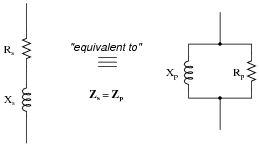We may write an equation for the impedance of each network in rectangular form, like this:

 $$Z_s=R_s+jX_s$$ (series network)

 $$Z_p= \frac{1}{\frac{1}{R_p}-j \frac{1}{X_p}}$$ (parallel network)

Since we are told these two networks are equivalent to one another, with equal impedances, these two expressions in rectangular form must also be equal to each other:

 $$R_s+jX_s= \frac{1}{\frac{1}{R_p}-j \frac{1}{X_p}}$$

Algebraically reduce this equation to its simplest form, showing how Rs, Rp, Xs, and Xp relate.

Challenge question: combine the result of that simplification with the equations solving for scalar impedance of series and parallel networks ($$Z^2_s= R^2_s+X^2_s$$ for series and $$Z^2_p= \frac{1}{\frac{1}{R^2_p}+\frac{1}{X^2_p}}$$ for parallel) to prove the following transformative equations, highly useful for “translating” a series network into a parallel network and visa-versa:

 $$Z^2=R_pR_s$$

 $$Z^2=X_pX_s$$

• #### Question 14

Determine the phase shift of the output voltage (Vout) with reference to the source voltage (0o) for each of the two switch positions, assuming the source frequency is such that XC = R:Note: you should be able to do all the necessary math mentally, without the aid of a calculating device!

• #### Question 15

This interesting bridge circuit is a variable phase-shifter. It works best when the excitation frequency is such that XC = R in each arm of the bridge: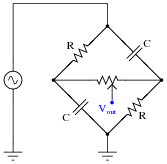Supposing that XC does equal R in each arm of the bridge, and that the potentiometer resistance is sufficiently high to limit current through it to a negligible level (in other words, Rpot >> R). Calculate the phase shift of Vout with respect to the excitation source voltage when:

The potentiometer wiper is fully left:
The potentiometer wiper is fully right:
The potentiometer wiper is perfectly centered:
• #### Question 16

This phase-shifting bridge circuit is supposed to provide an output voltage with a variable phase shift from -45o (lagging) to 45o (leading), depending on the position of the potentiometer wiper: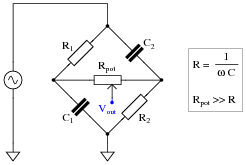Suppose, though, that there is a solder “bridge” between the terminals of resistor R1 on the circuit board. What effect will this fault have on the output of the circuit? Be as complete as you can in your answer.

• #### Question 17

This phase-shifting bridge circuit is supposed to provide an output voltage with a variable phase shift from -45o (lagging) to 45o (leading), depending on the position of the potentiometer wiper: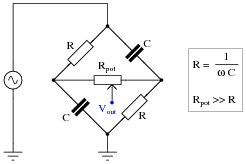Suppose, though, that the output signal is stuck at 45o leading the source voltage, no matter where the potentiometer is set. Identify a likely failure that could cause this to happen, and explain why this failure could account for the circuit’s strange behavior.

• #### Question 18

This phase-shifting bridge circuit is supposed to provide an output voltage with a variable phase shift from -45o (lagging) to 45o (leading), depending on the position of the potentiometer wiper: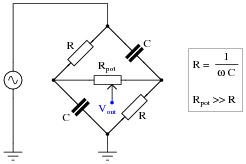Suppose, though, that the output signal registers as it should with the potentiometer wiper fully to the right, but diminishes greatly in amplitude as the wiper is moved to the left, until there is practically zero output voltage at the full-left position. Identify a likely failure that could cause this to happen, and explain why this failure could account for the circuit’s strange behavior.

• #### Question 19

Complex quantities may be expressed in either rectangular or polar form. Mathematically, it does not matter which form of expression you use in your calculations.

However, one of these forms relates better to real-world measurements than the other. Which of these mathematical forms (rectangular or polar) relates more naturally to measurements of voltage or current, taken with meters or other electrical instruments? For instance, which form of AC voltage expression, polar or rectangular, best correlates to the total voltage measurement in the following circuit?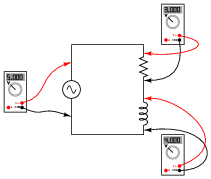• #### Question 20

Why are polarity marks ( and -) shown at the terminals of the components in this AC network?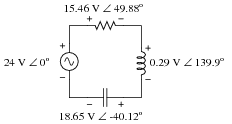Are these polarity markings really necessary? Do they make any sense at all, given the fact that AC by its very nature has no fixed polarity (because polarity alternates over time)? Explain your answer.

• #### Question 21

You should know that the line voltage of a three-phase, Y-connected, balanced system is always greater than the phase voltage by a factor of √3.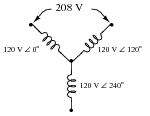Apply Kirchhoff’s Voltage Law (KVL) to the upper “loop” in this Y-connected alternator schematic to prove how 120 V ∠ 0o and 120 V ∠ 120o makes 208 V. Show the “polarity” marks for each of the voltages as part of your answer.

• #### Question 22

Draw a phasor diagram of the voltages in this Delta-connected polyphase system, and use trigonometry to calculate the voltage between points G and B.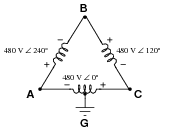• #### Question 23

Calculate the load impedance “seen” by the source, through the ideal transformer with a winding turns ratio of 4:1.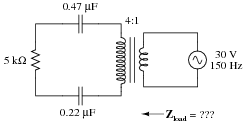• #### Question 24

Calculate all voltage drops in this circuit, expressing your answers in complex (polar) form: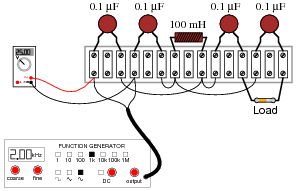The load resistor’s color code is as follows:

Brown, Black, Black, Brown, Violet

Assume the resistor’s error is 0%. That is, its resistance value is precisely equal to what the “digit” and “multiplier” color bands declare. The signal generator’s output is 25 volts RMS, at a frequency of 2 kHz.

Challenge question: what practical function does this circuit perform?